# 使用Pytorch实现NLP深度学习

PyTorch简介

Torch的张量（tensor）库简介

Softmax与概率

PyTorch中使用词嵌入

例子：N-Gram语言模型

Bi-LSTM条件随机场讨论

# PyTorch简介

## Torch的张量（tensor）库简介

import torch
import torch.nn as nn
import torch.nn.functional as F
import torch.optim as optim

torch.manual_seed(1)#设置随机种子，可以保证多次运行代码的运行结果一致

### 创建张量

import torch

V_data=[1.,2,3]
V=torch.Tensor(V_data)
print(V)

M_data=[[1.,2,3],[4,5,6]]
M=torch.Tensor(M_data)
print(M)

T_data=[[[1.,2],[3,4]],[[5,6],[7,8]]]
T=torch.Tensor(T_data)
print(T)

tensor([ 1.,  2.,  3.])
tensor([[ 1.,  2.,  3.],
[ 4.,  5.,  6.]])
tensor([[[ 1.,  2.],
[ 3.,  4.]],

[[ 5.,  6.],
[ 7.,  8.]]])

3维的张量是什么？这样想。如果你有一个向量，它的索引返回一个标量。如果你有一个矩阵，它的索引返回一个向量。而对于一个3维的张量，它的索引返回一个矩阵！

print(V)
print(M)
print(T)

tensor(1.)
tensor([ 1.,  2.,  3.])
tensor([[ 1.,  2.],
[ 3.,  4.]])


x=torch.randn((3,4,5))
print(x)

tensor([[[-1.5256, -0.7502, -0.6540, -1.6095, -0.1002],
[-0.6092, -0.9798, -1.6091, -0.7121,  0.3037],
[-0.7773, -0.2515, -0.2223,  1.6871,  0.2284],
[ 0.4676, -0.6970, -1.1608,  0.6995,  0.1991]],

[[ 0.8657,  0.2444, -0.6629,  0.8073,  1.1017],
[-0.1759, -2.2456, -1.4465,  0.0612, -0.6177],
[-0.7981, -0.1316,  1.8793, -0.0721,  0.1578],
[-0.7735,  0.1991,  0.0457,  0.1530, -0.4757]],

[[-0.1110,  0.2927, -0.1578, -0.0288,  0.4533],
[ 1.1422,  0.2486, -1.7754, -0.0255, -1.0233],
[-0.5962, -1.0055,  0.4285,  1.4761, -1.7869],
[ 1.6103, -0.7040, -0.1853, -0.9962, -0.8313]]])

### 张量的运算

x=torch.tensor([1,2,3])
y=torch.tensor([4,5,6])
z=x+y
print(z)

tensor([ 5,  7,  9])

https://pytorch.org/docs/stable/torch.html

#By default, concatenates rows
x_1=torch.randn(2,5)
y_1=torch.randn(3,5)
z_1=torch.cat([x_1,y_1])
print(z_1)

#concatenate columns
x_2=torch.randn(2,3)
y_2=torch.randn(2,2)
z_2=torch.cat([x_2,y_2],1)
print(z_2)

tensor([[ 0.6261, -1.1846, -0.5436,  0.6546, -0.5604],
[ 1.8735, -1.3139,  0.1034, -0.0350, -0.5010],
[-0.6748,  0.5247,  0.6635, -0.5871, -0.0938],
[ 0.2649, -0.7554,  2.2387, -0.0361,  0.6611],
[-1.7702,  1.5020,  0.7583, -0.2352,  1.3911]])
tensor([[-0.2167,  1.3546, -0.7955,  0.2682, -0.6354],
[-1.1214, -0.0510,  0.6120,  0.3620, -0.0748]])

### 改变张量的形状

x=torch.randn(2,3,4)
print(x)
print(x.view(2,12))
print(x.view(2,-1))

tensor([[[ 2.0470, -1.1800,  0.6039,  1.3999],
[ 0.8518,  0.3985,  0.1703, -0.4964],
[-0.0290,  0.5847,  0.1747,  0.2283]],

[[ 0.9133, -0.2119,  0.4301, -0.3655],
[ 0.2293, -2.0084,  1.2117, -0.5215],
[ 0.6462, -0.6679, -1.0030,  0.2034]]])
tensor([[ 2.0470, -1.1800,  0.6039,  1.3999,  0.8518,  0.3985,  0.1703,
-0.4964, -0.0290,  0.5847,  0.1747,  0.2283],
[ 0.9133, -0.2119,  0.4301, -0.3655,  0.2293, -2.0084,  1.2117,
-0.5215,  0.6462, -0.6679, -1.0030,  0.2034]])
tensor([[ 2.0470, -1.1800,  0.6039,  1.3999,  0.8518,  0.3985,  0.1703,
-0.4964, -0.0290,  0.5847,  0.1747,  0.2283],
[ 0.9133, -0.2119,  0.4301, -0.3655,  0.2293, -2.0084,  1.2117,
-0.5215,  0.6462, -0.6679, -1.0030,  0.2034]])

## 计算图和自动微分

x=torch.tensor([1.,2,3],requires_grad=True)
z=x+y
print(z)
print(z.grad_fn)

tensor([5., 7., 9.], grad_fn=<ThAddBackward>)
<ThAddBackward object at 0x0000018EB77D8160>

s=z.sum()
print(s)
print(s.grad_fn)

tensor(21., grad_fn=<SumBackward0>)
<SumBackward0 object at 0x00000228FFEB8128>

s知道它是由z的求和操作产生的，z知道它是x+y的和。所以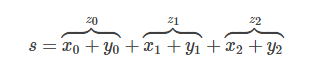s.backward()
print(x.grad)

tensor([ 1,  1,  1])

x=torch.randn(2,2)
y=torch.randn(2,2)
z=x+y
z=x+y
new_z=z.detach()
print(new_z.grad_fn)

False False
None
True
None

print(x.requires_grad)

print((x**2).requires_grad)

True
True
False

# 使用PyTorch进行深度学习

## 深度学习构建模块：仿射变换，非线性和目标函数

### 仿射变换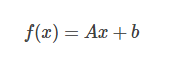PyTorch和其他深度学习框架做的事情和传统的代数学有所不同。它变换输入的一行而不是列。就是说，输出的第i行是输入的第i行经过A的变换（或映射），再加上偏置项。看下面的例子。

import torch
import torch.nn as nn
import torch.nn.functional as F
import torch.optim as optim

torch.manual_seed(1)
lin=nn.Linear(5,3)#maps from R^5 to R^3
data=torch.randn(2,5)
print(lin(data))

tensor([[ 0.1755, -0.3268, -0.5069],
[-0.6602,  0.2260,  0.1089]])

### 非线性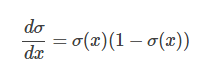data=torch.randn(2,2)
print(data)
print(F.relu(data))

tensor([[-0.5404, -2.2102],
[ 2.1130, -0.0040]])
tensor([[ 0.0000,  0.0000],
[ 2.1130,  0.0000]])

### Softmax与概率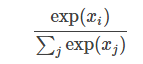data=torch.randn(5)
print(data)
print(F.softmax(data,dim=0))
print(F.softmax(data,dim=0).sum())#sums to 1
print(F.log_softmax(data,dim=0))#log e softmax

tensor([ 1.3800, -1.3505,  0.3455,  0.5046,  1.8213])
tensor([ 0.2948,  0.0192,  0.1048,  0.1228,  0.4584])
tensor(1.)
tensor([-1.2214, -3.9519, -2.2560, -2.0969, -0.7801])

## 优化和训练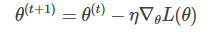## 在PyTorch中创建网络组件

### 举例：逻辑回归词袋分类器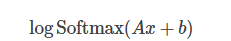data = [("me gusta comer en la cafeteria".split(), "SPANISH"),
("Give it to me".split(), "ENGLISH"),
("No creo que sea una buena idea".split(), "SPANISH"),
("No it is not a good idea to get lost at sea".split(), "ENGLISH")]
test_data = [("Yo creo que si".split(), "SPANISH"),
("it is lost on me".split(), "ENGLISH")]
word_to_ix = {}

for sent, _ in data + test_data:
for word in sent:
if word not in word_to_ix:
word_to_ix[word] = len(word_to_ix)
print(word_to_ix)

VOCAB_SIZE = len(word_to_ix)
NUM_LABELS = 2

class BoWClassifier(nn.Module):
def __init__(self, num_labels, vocab_size):
super(BoWClassifier, self).__init__()
self.linear = nn.Linear(vocab_size, num_labels)

def forward(self, bow_vec):
return F.log_softmax(self.linear(bow_vec), dim=1)

def make_bow_vector(sentence, word_to_ix):
vec = torch.zeros(len(word_to_ix))
for word in sentence:
ix = word_to_ix[word]
vec[ix] += 1
return vec.view(1, -1)

def make_target(label, label_to_ix):

model = BoWClassifier(NUM_LABELS, VOCAB_SIZE)

for param in model.parameters():
print(param)

sample = data
bow_vector = make_bow_vector(sample, word_to_ix)
log_probs = model(bow_vector)
print(log_probs)


{'en': 3, 'No': 9, 'buena': 14, 'it': 7, 'at': 22, 'sea': 12, 'cafeteria': 5, 'Yo': 23, 'la': 4, 'to': 8, 'creo': 10, 'is': 16, 'a': 18, 'good': 19, 'get': 20, 'idea': 15, 'que': 11, 'not': 17, 'me': 0, 'on': 25, 'gusta': 1, 'lost': 21, 'Give': 6, 'una': 13, 'si': 24, 'comer': 2}
Parameter containing:
tensor([[ 0.1194,  0.0609, -0.1268,  0.1274,  0.1191,  0.1739, -0.1099,
-0.0323, -0.0038,  0.0286, -0.1488, -0.1392,  0.1067, -0.0460,
0.0958,  0.0112,  0.0644,  0.0431,  0.0713,  0.0972, -0.1816,
0.0987, -0.1379, -0.1480,  0.0119, -0.0334],
[ 0.1152, -0.1136, -0.1743,  0.1427, -0.0291,  0.1103,  0.0630,
-0.1471,  0.0394,  0.0471, -0.1313, -0.0931,  0.0669,  0.0351,
-0.0834, -0.0594,  0.1796, -0.0363,  0.1106,  0.0849, -0.1268,
-0.1668,  0.1882,  0.0102,  0.1344,  0.0406]])
Parameter containing:
tensor([ 0.0631,  0.1465])
tensor([[-0.5378, -0.8771]])

label_to_ix={"SPANISH":0,"ENGLISH":1}

# before train, just to see a before-and-after
for instance, label in test_data:
bow_vector = make_bow_vector(instance, word_to_ix)
log_probs = model(bow_vector)
print(log_probs)
# before train, parameter value
print(next(model.parameters())[:, word_to_ix['creo']])

loss_function = nn.NLLLoss()
optimizer = optim.SGD(model.parameters(), lr=0.1)

# Usually, between 5 and 30 epochs is reasonable.
for epoch in range(100):
for instance, label in data:
bow_vec = make_bow_vector(instance, word_to_ix)
target = make_target(label, label_to_ix)
log_probs = model(bow_vec)
loss = loss_function(log_probs, target)
loss.backward()
optimizer.step()
for instance, label in test_data:
bow_vec = make_bow_vector(instance, word_to_ix)
log_probs = model(bow_vec)
print(log_probs)
print(next(model.parameters())[:, word_to_ix['creo']])

tensor([[-0.9297, -0.5020]])
tensor([[-0.6388, -0.7506]])
tensor([-0.1488, -0.1313])
tensor([[-0.2093, -1.6669]])
tensor([[-2.5330, -0.0828]])
tensor([ 0.2803, -0.5605])

# 词嵌入(Word Embeddings)：对词汇的语义进行编码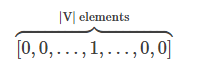one-hot编码方法很简单，但是它有两个巨大的缺点：

1. 它假设每个单词是独立的，互不相关。
2. 词向量长度巨大。

• The mathematician ran to the store.
• The physicist ran to the store.
• The mathematician solved the open problem.

• The physicist solved the open problem.

• 我们已经看到mathematician和physicist在句子中扮演了相同的角色。所以，它们应该有某种关系。
• 新句子中physicist的角色替换为mathematician，我们之前就看到过。

## 获取密集词嵌入（dense word embedding）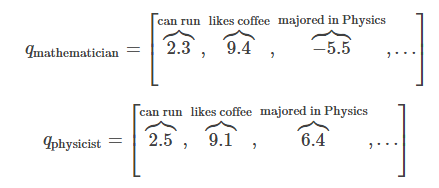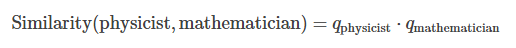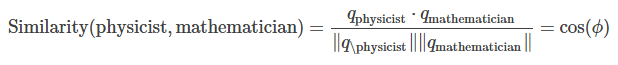## PyTorch中使用词嵌入

import torch
import torch.nn as nn
import torch.nn.functional as F
import torch.optim as optim

torch.manual_seed(1)
word_to_ix = {"hello": 0, "world": 1}
embeds = nn.Embedding(2, 5)  # 2words in vocab,5 dimensional embeddings
lookup_tensor = torch.tensor([word_to_ix['hello']], dtype=torch.long)
hello_embeds = embeds(lookup_tensor)
print(hello_embeds)

tensor([[ 0.6614,  0.2669,  0.0617,  0.6213, -0.4519]])

## 例子：N-Gram语言模型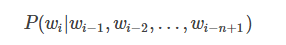CONTEXT_SIZE = 2
EMBEDDING_DIM = 10
test_sentence = """When forty winters shall besiege thy brow,
And dig deep trenches in thy beauty's field,
Thy youth's proud livery so gazed on now,
Will be a totter'd weed of small worth held:
Then being asked, where all thy beauty lies,
Where all the treasure of thy lusty days;
To say, within thine own deep sunken eyes,
Were an all-eating shame, and thriftless praise.
How much more praise deserv'd thy beauty's use,
If thou couldst answer 'This fair child of mine
Shall sum my count, and make my old excuse,'
Proving his beauty by succession thine!
This were to be new made when thou art old,
And see thy blood warm when thou feel'st it cold.""".split()
# build a list of tuples. Each tuple is ([word_i-2, word_i-1], target word)
trigrams = [([test_sentence[i], test_sentence[i + 1]], test_sentence[i + 2])
for i in range(len(test_sentence) - 2)]

print(trigrams[:3])
vocab = set(test_sentence)
word_to_ix = {word: i for i, word in enumerate(vocab)}

class NGramLanguageModeler(nn.Module):
def __init__(self, vocab_size, embedding_dim, context_size):
super(NGramLanguageModeler, self).__init__()
self.embeddings = nn.Embedding(vocab_size, embedding_dim)
self.linear1 = nn.Linear(context_size * embedding_dim, 128)
self.linear2 = nn.Linear(128, vocab_size)

def forward(self, inputs):
embeds = self.embeddings(inputs).view((1, -1))
out = F.relu(self.linear1(embeds))
out = self.linear2(out)
log_probs = F.log_softmax(out, dim=1)
return log_probs

losses = []
loss_function = nn.NLLLoss()
model = NGramLanguageModeler(len(vocab), EMBEDDING_DIM, CONTEXT_SIZE)
optimizer = optim.SGD(model.parameters(), lr=0.001)

for epoch in range(10):
total_loss = 0
for context, target in trigrams:
context_idxs = torch.tensor([word_to_ix[w] for w in context], dtype=torch.long)
log_probs = model(context_idxs)
loss = loss_function(log_probs, torch.tensor([word_to_ix[target]], dtype=torch.long))
loss.backward()
optimizer.step()
total_loss += loss
losses.append(total_loss)
print(losses)

[(['When', 'forty'], 'winters'), (['forty', 'winters'], 'shall'), (['winters', 'shall'], 'besiege')]
[tensor(518.5248), tensor(515.9546), tensor(513.4025), tensor(510.8652), tensor(508.3428), tensor(505.8335), tensor(503.3389), tensor(500.8579), tensor(498.3887), tensor(495.9313)]


## 练习：计算词嵌入：Continuous Bag-of-Words

Continuous Bag-of-Words（CBOW）模型经常在NLP深度学习中使用。它基于目标单词在上下文中前面的几个单词和后面的几个单词来预测单词。这和语言模型不同，因为它不是相继连接的一个序列，也不必须计算概率。通常情况下，CBOW用于快速的训练词嵌入向量，然后拿这些向量去初始化更复杂的模型。通常，这被称为预训练嵌入。它通常会提升几个百分点的性能。

CBOW模型表述如下。给你一个目标单词，和一个N（上下文前后单词的数量）。,...,,...,,把上下文单词的集合称为C，CBOW试图去最小化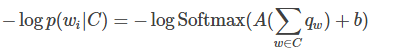• 思考下你需要定义什么参数
• 确保每个运算的形状具有合适的形状。你可以使用.view()方法来改变形状
CONTEXT_SIZE = 2  # 2 words to the left, 2 to the right
raw_text = """We are about to study the idea of a computational process.
Computational processes are abstract beings that inhabit computers.
As they evolve, processes manipulate other abstract things called data.
The evolution of a process is directed by a pattern of rules
called a program. People create programs to direct processes. In effect,
we conjure the spirits of the computer with our spells.""".split()

# By deriving a set from raw_text, we deduplicate the array
vocab = set(raw_text)
vocab_size = len(vocab)

word_to_ix = {word: i for i, word in enumerate(vocab)}
data = []
for i in range(2, len(raw_text) - 2):
context = [raw_text[i - 2], raw_text[i - 1],
raw_text[i + 1], raw_text[i + 2]]
target = raw_text[i]
data.append((context, target))
print(data[:5])

class CBOW(nn.Module):

def __init__(self):
pass

def forward(self, inputs):
pass

def make_context_vector(context, word_to_ix):
idxs = [word_to_ix[w] for w in context]

make_context_vector(data, word_to_ix)  # example

[(['We', 'are', 'to', 'study'], 'about'), (['are', 'about', 'study', 'the'], 'to'), (['about', 'to', 'the', 'idea'], 'study'), (['to', 'study', 'idea', 'of'], 'the'), (['study', 'the', 'of', 'a'], 'idea')]


CONTEXT_SIZE = 2  # 2 words to the left, 2 to the right
EMBEDDING_DIM=20
raw_text = """We are about to study the idea of a computational process.
Computational processes are abstract beings that inhabit computers.
As they evolve, processes manipulate other abstract things called data.
The evolution of a process is directed by a pattern of rules
called a program. People create programs to direct processes. In effect,
we conjure the spirits of the computer with our spells.""".split()

# By deriving a set from raw_text, we deduplicate the array
vocab = set(raw_text)
vocab_size = len(vocab)

word_to_ix = {word: i for i, word in enumerate(vocab)}
data = []
for i in range(2, len(raw_text) - 2):
context = [raw_text[i - 2], raw_text[i - 1],
raw_text[i + 1], raw_text[i + 2]]
target = raw_text[i]
data.append((context, target))
print(data[:5])

class CBOW(nn.Module):

def __init__(self,vocab_size,context_size,embedding_dim):
super(CBOW,self).__init__()
self.embeddings=nn.Embedding(vocab_size,embedding_dim)
self.linear1=nn.Linear(context_size*2*embedding_dim,256)
self.linear2=nn.Linear(256,vocab_size)

def forward(self, inputs):
embeds=self.embeddings(inputs).view(1,-1)
out=F.relu(self.linear1(embeds))
out=self.linear2(out)
out=F.log_softmax(out,dim=1)
return out

def make_context_vector(context, word_to_ix):
idxs = [word_to_ix[w] for w in context]

make_context_vector(data, word_to_ix)  # example
losses=[]
loss_function=nn.NLLLoss()
model=CBOW(vocab_size,CONTEXT_SIZE,EMBEDDING_DIM)
optimizer=optim.SGD(model.parameters(),lr=0.001)

for epoch in range(10):
total_loss=0
for context,target in data:
context_idxs=make_context_vector(context,word_to_ix)
log_probs1=model(context_idxs)
loss=loss_function(log_probs1,torch.tensor([word_to_ix[target]],dtype=torch.long))
total_loss += loss
loss.backward()
optimizer.step()
losses.append(total_loss)

print(losses)

[tensor(226.9995), tensor(224.1626), tensor(221.3551), tensor(218.5737), tensor(215.8168), tensor(213.0830), tensor(210.3716), tensor(207.6829), tensor(205.0123), tensor(202.3596)]


# 序列模型和LSTM（Long-Short Term Memory）网络

## 在PyTorch中使用LSTM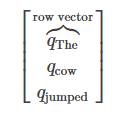import torch
import torch.nn as nn
import torch.nn.functional as F
import torch.optim as optim

torch.manual_seed(1)
lstm=nn.LSTM(3,4)#Iput dim is 3, output dim is 4
inputs=[torch.randn(1,3) for _ in range(5)]#make a sequence of length

#initialize the hidden state
hidden=(torch.randn(1,1,4),torch.randn(1,1,4))

for i in inputs:
out,hidden=lstm(i.view(1,1,-1),hidden)

inputs=torch.cat(inputs).view(len(inputs),1,-1)
hidden=(torch.randn(1,1,4),torch.randn(1,1,4))
out,hidden=lstm(inputs,hidden)
print(out)
print(hidden)


tensor([[[-0.2566,  0.1294,  0.0441, -0.5235]],

[[-0.4444,  0.0762,  0.0304, -0.3889]],

[[-0.1741,  0.1061, -0.0179, -0.1505]],

[[-0.1409,  0.1110,  0.0773, -0.2373]],

[[-0.2308,  0.1164, -0.0115, -0.2423]]])
(tensor([[[-0.2308,  0.1164, -0.0115, -0.2423]]]), tensor([[[-0.4093,  0.6065, -0.0288, -0.4107]]]))

## 例子：使用LSTM实现词性标注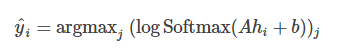def prepare_sequence(seq,to_ix):
idxs=[to_ix[w] for w in seq]

training_data = [
("The dog ate the apple".split(), ["DET", "NN", "V", "DET", "NN"]),
("Everybody read that book".split(), ["NN", "V", "DET", "NN"])
]
word_to_ix={}
for sent,tag in training_data:
for word in sent:
if word not in word_to_ix:
word_to_ix[word]=len(word_to_ix)
print(word_to_ix)

tag_to_ix={"DET":0,"NN":1,"V":2}
EMBEDDING_DIM=6
HIDDEN_DIM=6


{'Everybody': 5, 'ate': 2, 'apple': 4, 'that': 7, 'read': 6, 'dog': 1, 'book': 8, 'the': 3, 'The': 0}

class LSTMTagger(nn.Module):
def __init__(self,embedding_dim,hidden_dim,vocab_size,tagset_size):
super(LSTMTagger,self).__init__()
self.hidden_dim=hidden_dim
self.word_embeddings=nn.Embedding(vocab_size,embedding_dim)
self.lstm=nn.LSTM(embedding_dim,hidden_dim)
self.hidden2tag=nn.Linear(hidden_dim,tagset_size)
self.hidden=self.init_hidden()
def init_hidden(self):
#the axes semantics are (num_layers,minibatch_size,hidden_size)
return (torch.zeros(1,1,self.hidden_dim),
(torch.zeros(1,1,self.hidden_dim)))
def forward(self, sentence):
embeds=self.word_embeddings(sentence)
lstm_out,self.hidden=self.lstm(embeds.view(len(sentence),1,-1),self.hidden)
tag_space=self.hidden2tag(lstm_out.view(len(sentence),-1))
tag_scores=F.log_softmax(tag_space,dim=1)
return tag_scores

model=LSTMTagger(EMBEDDING_DIM,HIDDEN_DIM,len(word_to_ix),len(tag_to_ix))
loss_function=nn.NLLLoss()
optimizer=optim.SGD(model.parameters(),lr=0.1)
#before training
inputs=prepare_sequence(training_data,word_to_ix)
tag_scores=model(inputs)
print(tag_scores)

for epoch in range(300):
for sentence,tags in training_data:
model.hidden=model.init_hidden()
sentence_in=prepare_sequence(sentence,word_to_ix)
targets=prepare_sequence(tags,tag_to_ix)
tag_scores=model(sentence_in)
loss=loss_function(tag_scores,targets)
loss.backward()
optimizer.step()
#after training
inputs=prepare_sequence(training_data,word_to_ix)
tag_scores=model(inputs)
print(tag_scores)

tensor([[-0.9672, -1.1054, -1.2421],
[-0.9457, -1.2572, -1.1174],
[-0.9538, -1.1928, -1.1669],
[-0.9761, -1.0899, -1.2483],
[-0.9606, -1.1283, -1.2249]])
tensor([[-0.0773, -3.7290, -2.9876],
[-5.2341, -0.0289, -3.7640],
[-2.4058, -2.8015, -0.1636],
[-0.0431, -5.0451, -3.3325],
[-5.8218, -0.0123, -4.6852]])


## 练习：使用字符级特征增强LSTM词性标注器

• 在你的模型中将有两个LSTM。原来的那个输出标签分数，新的输出单词字符级表述。
• 要在字符上执行序列模型，你将使用字符嵌入。字符的嵌入向量将为字符LSTM的输入。

# 高级：动态决策和Bi-LSTM CRF

## 动态与静态深度学习工具包

PyTorch是一个动态神经网络工具包。另一个动态工具包是Dynet（我提到它因为PyTorch和Dynet很相似。如果你看到Dynet的一个例子，很可能会帮助你在PyTorch中实现它）。与之相反的是静态工具包，其中包括Theano，Keras，TensorFlow等。核心的不同如下所述：

• 在静态工具包中，你定义计算图一次，编译它，然后传递实例运行
• 在动态工具包中，你为每个实例定义一次计算图。它从不编译并在运行时执行。

• 自底而上构建分析树
• 标记根节点（句子的单词）
• 使用神经网络和词嵌入来寻找成分的组合。每当你形成新的成分时，使用某种技术来获得成分的嵌入。在这种情况下，我们的网络结构完全依赖于输入语句。句子“The green cat scratched the wall”，在模型的某个点上，我们想要使用组合（i，j，r）=（1,3，NP）（也就是说，一个NP，noun phrase即名词陈芬跨越单词1-单词3，在本例中是“the green cat”）

## Bi-LSTM条件随机场讨论

1. 写下标签k在i步维特比变量的循环
2. 修改上面的循环来计算前向变量
3. 再次修改上述循环来计算对数空间的前向变量（提示：log-sum-exp）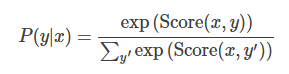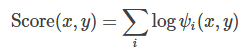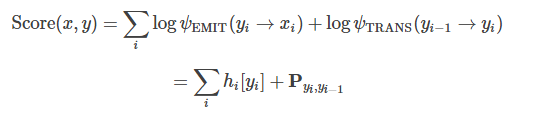## 练习：判别标注的一种新的损失函数

©️2019 CSDN 皮肤主题: 大白 设计师: CSDN官方博客